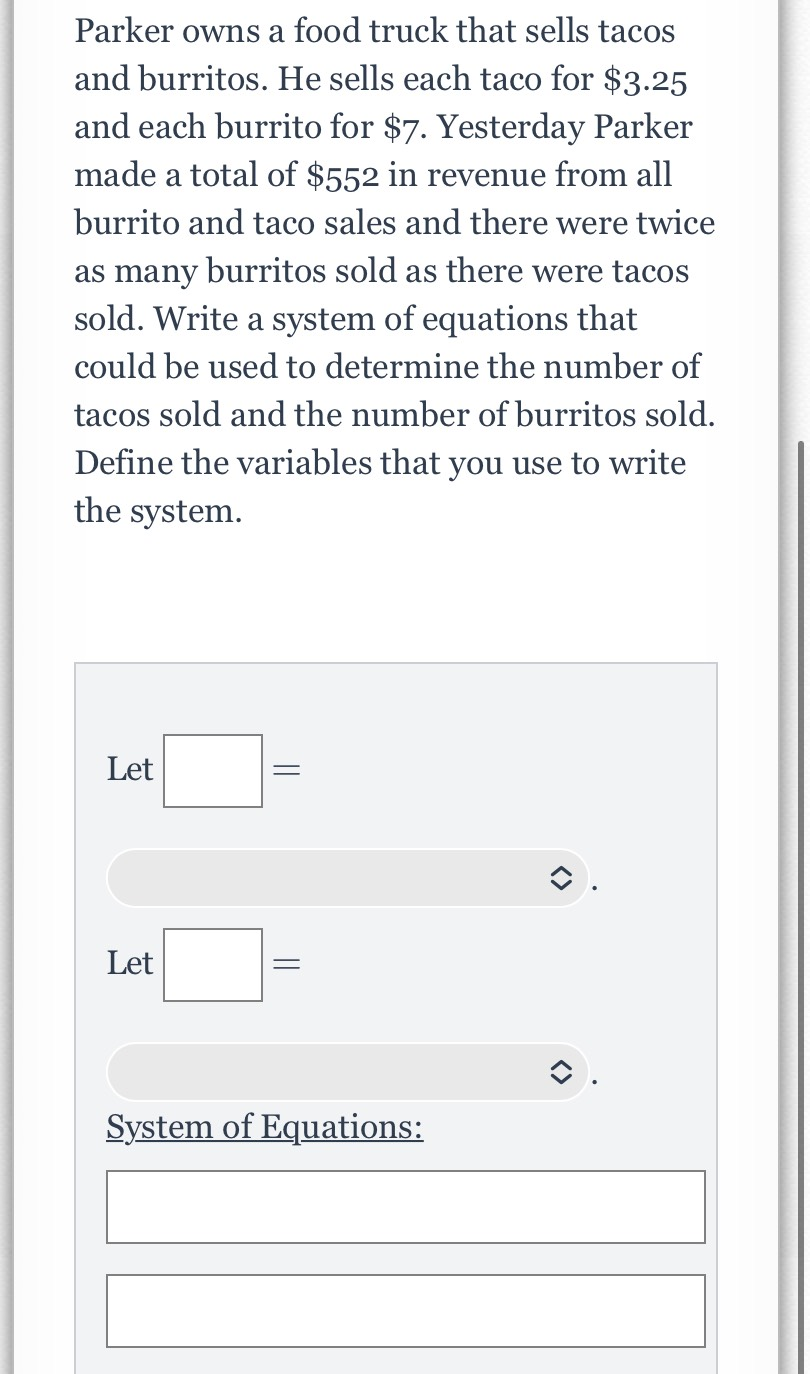### Still have math questions?

Algebra
QuestionParker owns a food truck that sells tacos and burritos. He sells each taco for $$\ 3.25$$

and each burrito for $$\ 7$$ . Yesterday Parker made a total of $$\ 552$$ in revenue from all burrito and taco sales and there were twice as many burritos sold as there were tacos sold. Write a system of equations that could be used to determine the number of tacos sold and the number of burritos sold. Define the variables that you use to write the system. System of Equations: Let $$=$$